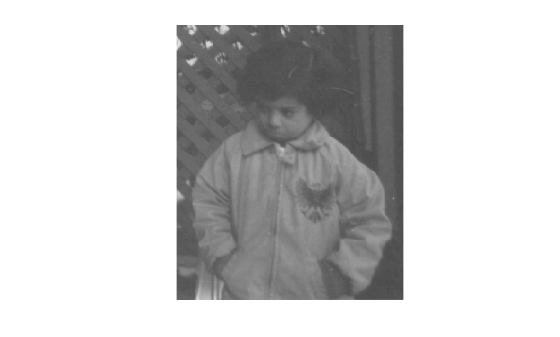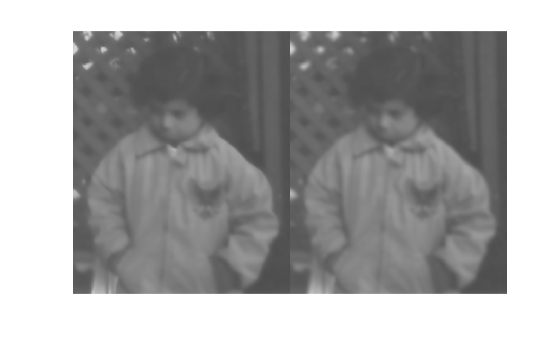Documentation

# imguidedfilter

Guided filtering of images

## Syntax

``B = imguidedfilter(A,G)``
``B = imguidedfilter(A)``
``B = imguidedfilter(___,Name,Value)``

## Description

````B = imguidedfilter(A,G)` filters binary, grayscale, or RGB image `A` using the guided filter, where the filtering process is guided by image `G`.```

example

````B = imguidedfilter(A)` filters input image `A` under self-guidance, using `A` itself as the guidance image. This can be used for edge-preserving smoothing of image `A`.```
````B = imguidedfilter(___,Name,Value)` filters the image `A` using name-value pairs to control aspects of guided filtering.```

## Examples

collapse all

```A = imread('pout.tif'); imshow(A)```Smooth the image using `imguidedfilter`. In this syntax, `imguidedfilter` uses the image itself as the guidance image.

`Iguided = imguidedfilter(A);`

For comparison, smooth the original image using a Gaussian filter defined by `imgaussfilt`. Set the standard deviation of the filter to 2.5 so that the degree of smoothing approximately matches that of the guided filter.

`Igaussian = imgaussfilt(A,2);`

Display the result of guided filtering and the result of Gaussian filtering. Observe that the flat regions of the two filtered images, such as the jacket and the face, have similar amounts of smoothing. However, the guided filtered image better preserves the sharpness of edges, such as around the trellis and the collar of the white shirt.

`montage({Iguided,Igaussian})`## Input Arguments

collapse all

Image to be filtered, specified as a binary, grayscale, or RGB image.

Data Types: `single` | `double` | `int8` | `int16` | `int32` | `uint8` | `uint16` | `uint32` | `logical`

Image to use as a guide during filtering, specified as a binary, grayscale, or RGB image of the same size as image `A`.

Data Types: `single` | `double` | `int8` | `int16` | `int32` | `uint8` | `uint16` | `uint32` | `logical`

### Name-Value Pair Arguments

Specify optional comma-separated pairs of `Name,Value` arguments. `Name` is the argument name and `Value` is the corresponding value. `Name` must appear inside quotes. You can specify several name and value pair arguments in any order as `Name1,Value1,...,NameN,ValueN`.

Example: ```Ismooth = imguidedfilter(A,'NeighborhoodSize',[4 4]);```

Size of the rectangular neighborhood around each pixel used in guided filtering, specified as a positive integer or a 2-element vector of positive integers. If you specify a scalar value, such as `Q`, then the neighborhood is a square of size ```[Q Q]```. Do not specify a value greater than the size of the image.

Example: ```Ismooth = imguidedfilter(A,'NeighborhoodSize',[7 7]);```

Data Types: `single` | `double` | `int8` | `int16` | `int32` | `int64` | `uint8` | `uint16` | `uint32` | `uint64`

Amount of smoothing in the output image, specified as a positive number. If you specify a small value, only neighborhoods with small variance (uniform areas) will get smoothed and neighborhoods with larger variance (such as around edges) will not be smoothed. If you specify a larger value, high variance neighborhoods, such as stronger edges, will get smoothed in addition to the relatively uniform neighborhoods. Start with the default value, check the results, and adjust the default up or down to achieve the effect you desire.

If you specify a guide image `G`, then the default value of `degreeOfSmoothing` depends on the data type of `G`, and is calculated as `0.01*diff(getrangefromclass(G)).^2`. For example, the default degree of smoothing is `650.25` for images of data type `uint8`, and the default is `0.01` for images of data type `double` with pixel values in the range [0, 1]. If you do not specify a guide image, then the default value depends similarly on the data type of image `A`.

Example: ```Ismooth = imguidedfilter(A,'DegreeOfSmoothing',650.25);```

Data Types: `single` | `double` | `int8` | `int16` | `int32` | `int64` | `uint8` | `uint16` | `uint32` | `uint64`

## Output Arguments

collapse all

Filtered image, returned as a numeric array of the same size and data type as `A`

## Tips

• The parameter `DegreeOfSmoothing` specifies a soft threshold on variance for the given neighborhood. If a pixel's neighborhood has variance much lower than the threshold, it will see some amount of smoothing. If a pixel's neighborhood has variance much higher than the threshold it will have little to no smoothing.

• Input images `A` and `G` can be of different classes. If either `A` or `G` is of class integer or logical, then `imguidedfilter` converts them to floating-point precision for internal computation.

• Input images `A` and `G` can have different number of channels.

• If `A` is an `RGB` image and `G` is a grayscale or binary image, then `imguidedfilter` uses `G` for guidance for all the channels of `A` independently.

• If both `A` and `G` are RGB images, then `imguidedfilter` uses each channel of `G` for guidance for the corresponding channel of `A`, i.e. plane-by-plane behavior.

• If `A` is a grayscale or binary image and `G` is an RGB image, then `imguidedfilter` uses all the three channels of `G` for guidance (color statistics) for filtering `A`.

 Kaiming He, Jian Sun, Xiaoou Tang, Guided Image Filtering. IEEE Transactions on Pattern Analysis and Machine Intelligence, Volume 35, Issue 6, pp. 1397-1409, June 2013